# Induction Motor & Linear Induction Motors Formulas & Equations

## Formulas and Equations for Linear and Induction Motors

The following equations and formulas related to linear and induction motors can be used to calculate the basic parameters while analyzing and designing a single phase and three phase induction motor.### Formula and Equations for Induction Motor:

#### Induced EMF:

eind = vBl

where

• eind = induced EMF
• v = velocity of the rotor
• B = magnetic flux density
• l = length of conductors inside magnetic field

#### Torque Induced:

Terms used in Motor Torque Equations and formulas.

• Ns = Synchronous speed
• s = slip of the motor
• s= breakdown or pull-out slip
• E1 = stator voltage or input voltage
• E2 = Rotor EMF per phase at a standstill
• R2 = Rotor Resistance Per Phase
• X2 = Rotor Reactance Per Phase
• V = supply voltage
• K = rotor/stator turn ration per Phase

Starting Torque• Maximum Starting Torque Condition

R= X2

• Starting Torque Relation With Supply Voltage

Tst  α  V2

• Torque In Running Condition

• Gross Torque• Maximum Running Torque Condition

R= sX2

• Maximum Running Torque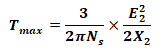• Breakdown Slip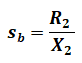• Torque Relation With Max Torque#### Slip Speed & Slip of Induction Motor:

Slip speed is the difference between synchronous speed and rotor speed;

• Nslip = N­– N­                         (Speed in RPM)
• ωslip = ω– ω­                        (Angular speed in Rad/sec)

Where

• N­­slip = Slip speed
• Ns ­= Synchronous speed = 120f/P
• N­­ = Rotor speed of motor

The slip of induction motor is a relative term expressed in percentage. It is given by: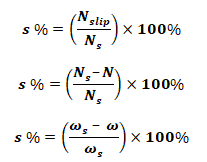Where

#### Rotor Speed:

The rotor speed of induction motor is given by

• N = (1-s)N               (Speed in RPM)
• ω = (1-s) ω s             (Angular speed in Rad/sec)

#### Electrical Frequency on the Rotor: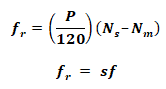Where

• f = Rotor Frequency
• f  = Line Frequency
• P = Number of Poles

#### Power of Induction Motor:

Related terms used in Motor Power Formulas and Equation.

• P1 = Stator input Power
• P2 = Rotor Input power
• Pm = Rotor Gross Output Power
• Pout = Output Power
• Tg = gross torque
• Tsh = shaft torque

Rotor Input Power:

P2 = Tgωs

• Rotor Gross Output Power:

Pm = Tgω

• Output Power:

Pout = Tshω

P1 = P2 + stator Losses = P+ Rotor Copper Losses = Pout + Windage & friction LossesRotor Input Power: Output Mechanical Power: Rotor Cu loss ratio:Where

• Pcr = I2R = rotor Copper loss

#### Synchronous Watt:

The torque at which the machine at synchronous speed will generate one watt;#### Efficiency of Induction Motor:

• Rotor Efficiency:• Overall Efficiency### Formula & Equations for Linear Induction Motor:

#### Synchronous Speed:

• v = linear synchronous speed
• w = width of one pole-pitch
• f = line frequency

#### Slip:Where

• v= linear synchronous speed
• v = Actual speed

#### Thrust or Force:Where

P2 = Rotor input Power

#### Rotor Cu Loss: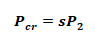#### Gross Mechanical Power:Related Formulas and Equations Posts: